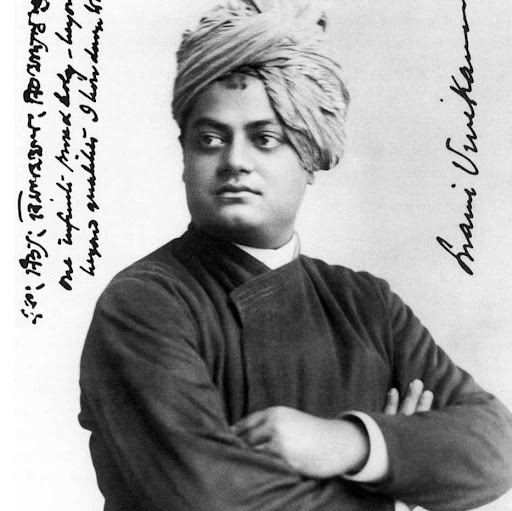5:03 AM
@JackRod Hi. Sorry I wasn't around much yesterday but I'm here this morning.

2 hours later…6:52 AM
@JohnRennie hi@JackRod Hisir yesterday postI have no idea how you write a differential equation for the internal energy in a Joule expansion. Sorry :-(no not in joule expansion
in general I was talking
in general know internal is function of temperature at constant volume is $c_v=Du/DT$
assume D as partial
using the equation $\deltaQ=du+dw$I guess you start with dU = dq + dw and proceed from there.6:57 AM
yes partially differentiating it
when volume is constant and when temperature is constantTo be honest my memory of thermodynamics is a bit vague these days so I'm not sure how much I can help.sorry for asking it but i have faith in u thermo is a topic which is my sem
please I am bit of confuse in this sectionIt would be better if we considered a specific problem you've been set. When we are just talking generally I'm not sure how to proceed.ok I have a problem
for a gas obeying Vanderwall gas equation change in internal energy with volume at constant temp given by?@JohnRennieI don't know, sorry :-(
That's going beyond what I can remember of my thermodynamics from university.7:08 AM
ooO
@JohnRennieSorry :-(
1Previous, I proved that $$\frac{T_2}{T_1}^{C_V/(C_p-C_V)}=\frac{V_1}{V_2}$$ I've been asked to derive a similar expression for a gas that obeys the equation of state $$p=\frac{RT}{(\overline{V}-b)}$$ I started with the first law and made the assumption that \mathrm dU=\frac{\partial{U}}{\p...

Does that help?I am sorry:) too sir.
@JohnRennie yes
@JohnRennie does sir satan is good at thermo is in phy major right?I think he is doing an engineering course, not physics, but you could try asking him.engineering in?
even I am doing engineering in phyI'm not sure what course he is doing ...7:20 AM
okThe problem is that many subjects in physics I only did for a year because I specialised into quantum chemistry in my last year. In particular I only did thermodynamics for a year and I didn't like it so I have forgotten most of it.
So while I can help with JEE level thermodynamics I will struggle when it comes to degree level questions.
And as you move on to the later years of your course you'll leave me far behind :-)